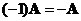## Scalar Multiple of a Matrix

When we multiply a real number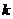with a matrix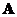, we have the scalar multiple of. The scalar multiple matrix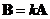has the same size of the input matrixand it is obtained by multiplying each element ofbythat is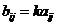.

Example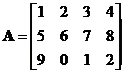The interactive program below shows you the result of matrix scalar multiple. Your input is a matrix of any size but less than 100 scalar elements and a scalar number. Random Example will generate both random matrix and random scalar.

Matrix A = scalar k =

## Properties

Some important properties of matrix scalar multiple are

• Matrix scalar multiple is a commutative operation. When the order is reversed, you will still get the same result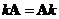• Matrix scalar multiple is an associative operation. You can exchange the order of computation (operation inside parentheses are to be computed first) does not change the result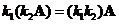.
• Matrix scalar multiple is a distributive operation. You can distribute (and group) the scalar with respect to addition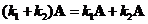and multiplication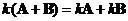.
• Matrix scalar multiple is also an associative operation with respect to matrix product . You can exchange the order of computation (operation inside parentheses are to be computed first) does not change the result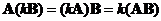.
• When the scalar is zero, matrix scalar multiple will produce a zero matrix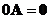• When the scalar is one, matrix scalar multiple will produce the input matrix itself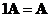• When the scalar is negative one, matrix scalar multiple will produce the negative of the input matrix